SNAP Library 6.0, Developer Reference  2020-12-09 16:24:20 SNAP, a general purpose, high performance system for analysis and manipulation of large networks
TNEGraph::TNodeI Class Reference

Node iterator. Only forward iteration (operator++) is supported. More...

`#include <graph.h>`

Collaboration diagram for TNEGraph::TNodeI: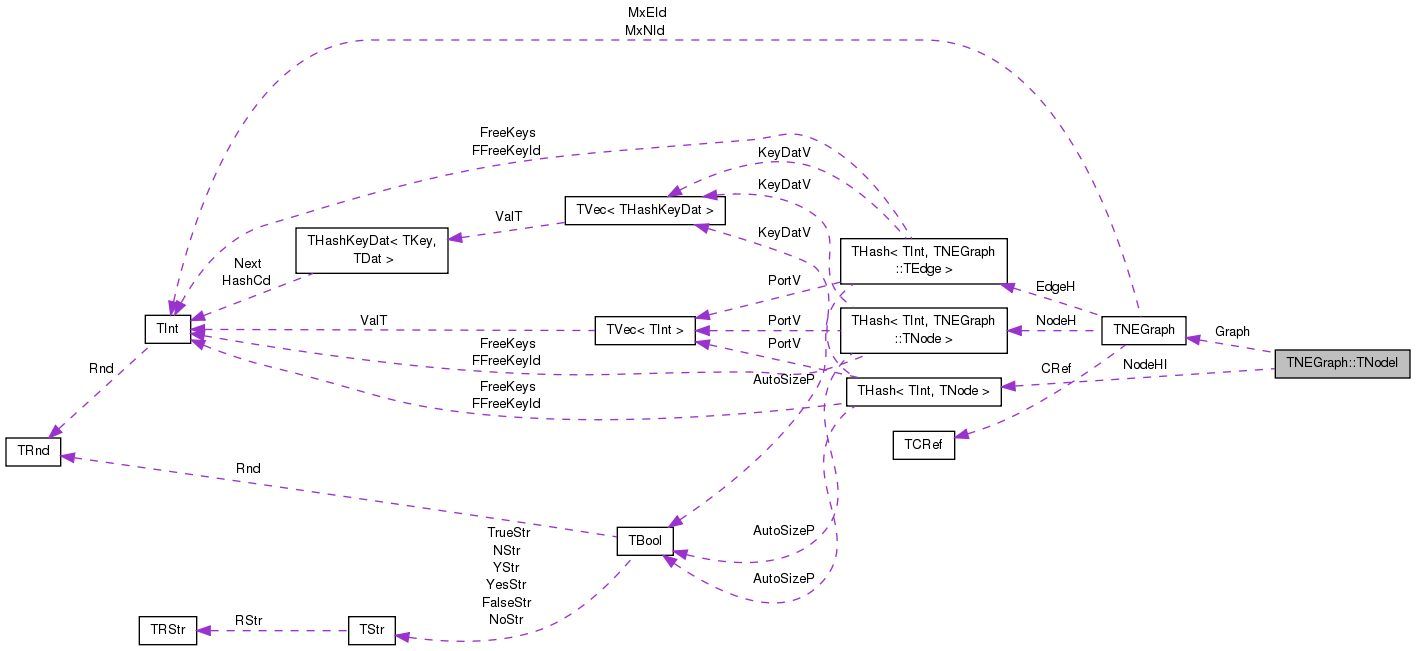[legend]

## Public Member Functions

TNodeI ()

TNodeI (const THashIter &NodeHIter, const TNEGraph *GraphPt)

TNodeI (const TNodeI &NodeI)

TNodeIoperator= (const TNodeI &NodeI)

TNodeIoperator++ (int)
Increment iterator. More...

TNodeIoperator-- (int)
Decrement iterator. More...

bool operator< (const TNodeI &NodeI) const

bool operator== (const TNodeI &NodeI) const

int GetId () const
Returns ID of the current node. More...

int GetDeg () const
Returns degree of the current node, the sum of in-degree and out-degree. More...

int GetInDeg () const
Returns in-degree of the current node. More...

int GetOutDeg () const
Returns out-degree of the current node. More...

int GetInNId (const int &EdgeN) const
Returns ID of EdgeN-th in-node (the node pointing to the current node). More...

int GetOutNId (const int &EdgeN) const
Returns ID of EdgeN-th out-node (the node the current node points to). More...

int GetNbrNId (const int &EdgeN) const
Returns ID of EdgeN-th neighboring node. More...

bool IsInNId (const int &NId) const
Tests whether node with ID NId points to the current node. More...

bool IsOutNId (const int &NId) const
Tests whether the current node points to node with ID NId. More...

bool IsNbrNId (const int &NId) const
Tests whether node with ID NId is a neighbor of the current node. More...

int GetInEId (const int &EdgeN) const
Returns ID of EdgeN-th in-edge. More...

int GetOutEId (const int &EdgeN) const
Returns ID of EdgeN-th out-edge. More...

int GetNbrEId (const int &EdgeN) const
Returns ID of EdgeN-th in or out-edge. More...

bool IsInEId (const int &EId) const
Tests whether the edge with ID EId is an in-edge of current node. More...

bool IsOutEId (const int &EId) const
Tests whether the edge with ID EId is an out-edge of current node. More...

bool IsNbrEId (const int &EId) const
Tests whether the edge with ID EId is an in or out-edge of current node. More...

## Private Types

typedef THash< TInt, TNode >::TIter THashIter

## Private Attributes

THashIter NodeHI

const TNEGraphGraph

class TNEGraph

## Detailed Description

Node iterator. Only forward iteration (operator++) is supported.

Definition at line 706 of file graph.h.

## Member Typedef Documentation

 typedef THash::TIter TNEGraph::TNodeI::THashIter
private

Definition at line 708 of file graph.h.

## Constructor & Destructor Documentation

 TNEGraph::TNodeI::TNodeI ( )
inline

Definition at line 712 of file graph.h.

712 : NodeHI(), Graph(NULL) { }
THashIter NodeHI
Definition: graph.h:709
const TNEGraph * Graph
Definition: graph.h:710
 TNEGraph::TNodeI::TNodeI ( const THashIter & NodeHIter, const TNEGraph * GraphPt )
inline

Definition at line 713 of file graph.h.

713 : NodeHI(NodeHIter), Graph(GraphPt) { }
THashIter NodeHI
Definition: graph.h:709
const TNEGraph * Graph
Definition: graph.h:710
 TNEGraph::TNodeI::TNodeI ( const TNodeI & NodeI )
inline

Definition at line 714 of file graph.h.

714 : NodeHI(NodeI.NodeHI), Graph(NodeI.Graph) { }
THashIter NodeHI
Definition: graph.h:709
const TNEGraph * Graph
Definition: graph.h:710

## Member Function Documentation

 int TNEGraph::TNodeI::GetDeg ( ) const
inline

Returns degree of the current node, the sum of in-degree and out-degree.

Definition at line 726 of file graph.h.

726 { return NodeHI.GetDat().GetDeg(); }
THashIter NodeHI
Definition: graph.h:709
 int TNEGraph::TNodeI::GetId ( ) const
inline

Returns ID of the current node.

Definition at line 724 of file graph.h.

724 { return NodeHI.GetDat().GetId(); }
THashIter NodeHI
Definition: graph.h:709

Here is the caller graph for this function: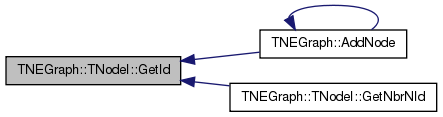int TNEGraph::TNodeI::GetInDeg ( ) const
inline

Returns in-degree of the current node.

Definition at line 728 of file graph.h.

728 { return NodeHI.GetDat().GetInDeg(); }
THashIter NodeHI
Definition: graph.h:709
 int TNEGraph::TNodeI::GetInEId ( const int & EdgeN ) const
inline

Returns ID of EdgeN-th in-edge.

Definition at line 752 of file graph.h.

752 { return NodeHI.GetDat().GetInEId(EdgeN); }
THashIter NodeHI
Definition: graph.h:709
 int TNEGraph::TNodeI::GetInNId ( const int & EdgeN ) const
inline

Returns ID of EdgeN-th in-node (the node pointing to the current node).

Range of NodeN: 0 <= NodeN < GetInDeg().

Definition at line 734 of file graph.h.

References TNEGraph::GetEdge().

734 { return Graph->GetEdge(NodeHI.GetDat().GetInEId(EdgeN)).GetSrcNId(); }
THashIter NodeHI
Definition: graph.h:709
TEdge & GetEdge(const int &EId)
Definition: graph.h:792
const TNEGraph * Graph
Definition: graph.h:710

Here is the call graph for this function: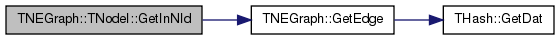int TNEGraph::TNodeI::GetNbrEId ( const int & EdgeN ) const
inline

Returns ID of EdgeN-th in or out-edge.

Definition at line 756 of file graph.h.

756 { return NodeHI.GetDat().GetNbrEId(EdgeN); }
THashIter NodeHI
Definition: graph.h:709
 int TNEGraph::TNodeI::GetNbrNId ( const int & EdgeN ) const
inline

Returns ID of EdgeN-th neighboring node.

Range of NodeN: 0 <= NodeN < GetNbrDeg().

Definition at line 742 of file graph.h.

742  { const TEdge& E = Graph->GetEdge(NodeHI.GetDat().GetNbrEId(EdgeN));
743  return GetId()==E.GetSrcNId() ? E.GetDstNId():E.GetSrcNId(); }
THashIter NodeHI
Definition: graph.h:709
TEdge & GetEdge(const int &EId)
Definition: graph.h:792
const TNEGraph * Graph
Definition: graph.h:710
int GetId() const
Returns ID of the current node.
Definition: graph.h:724

Here is the call graph for this function: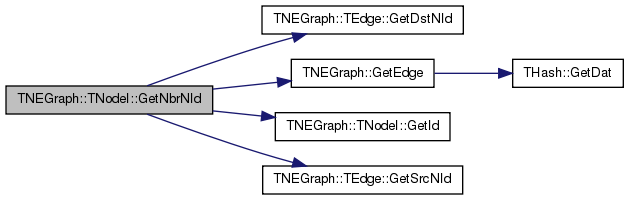int TNEGraph::TNodeI::GetOutDeg ( ) const
inline

Returns out-degree of the current node.

Definition at line 730 of file graph.h.

730 { return NodeHI.GetDat().GetOutDeg(); }
THashIter NodeHI
Definition: graph.h:709
 int TNEGraph::TNodeI::GetOutEId ( const int & EdgeN ) const
inline

Returns ID of EdgeN-th out-edge.

Definition at line 754 of file graph.h.

754 { return NodeHI.GetDat().GetOutEId(EdgeN); }
THashIter NodeHI
Definition: graph.h:709
 int TNEGraph::TNodeI::GetOutNId ( const int & EdgeN ) const
inline

Returns ID of EdgeN-th out-node (the node the current node points to).

Range of NodeN: 0 <= NodeN < GetOutDeg().

Definition at line 738 of file graph.h.

References TNEGraph::GetEdge().

738 { return Graph->GetEdge(NodeHI.GetDat().GetOutEId(EdgeN)).GetDstNId(); }
THashIter NodeHI
Definition: graph.h:709
TEdge & GetEdge(const int &EId)
Definition: graph.h:792
const TNEGraph * Graph
Definition: graph.h:710

Here is the call graph for this function: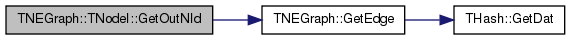bool TNEGraph::TNodeI::IsInEId ( const int & EId ) const
inline

Tests whether the edge with ID EId is an in-edge of current node.

Definition at line 758 of file graph.h.

Referenced by IsNbrEId().

758 { return NodeHI.GetDat().IsInEId(EId); }
THashIter NodeHI
Definition: graph.h:709

Here is the caller graph for this function: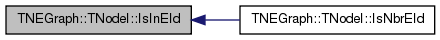bool TNEGraph::TNodeI::IsInNId ( const int & NId ) const

Tests whether node with ID NId points to the current node.

Definition at line 468 of file graph.cpp.

Referenced by IsNbrNId().

468  {
469  const TNode& Node = NodeHI.GetDat();
470  for (int edge = 0; edge < Node.GetInDeg(); edge++) {
471  if (NId == Graph->GetEdge(Node.GetInEId(edge)).GetSrcNId())
472  return true;
473  }
474  return false;
475 }
THashIter NodeHI
Definition: graph.h:709
TEdge & GetEdge(const int &EId)
Definition: graph.h:792
const TNEGraph * Graph
Definition: graph.h:710

Here is the call graph for this function: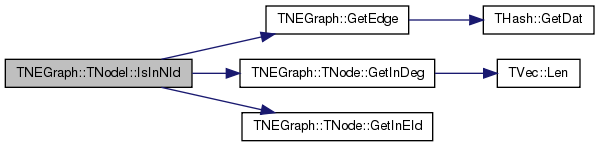Here is the caller graph for this function: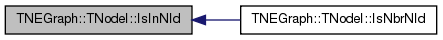bool TNEGraph::TNodeI::IsNbrEId ( const int & EId ) const
inline

Tests whether the edge with ID EId is an in or out-edge of current node.

Definition at line 762 of file graph.h.

References IsInEId(), and IsOutEId().

762 { return IsInEId(EId) || IsOutEId(EId); }
bool IsOutEId(const int &EId) const
Tests whether the edge with ID EId is an out-edge of current node.
Definition: graph.h:760
bool IsInEId(const int &EId) const
Tests whether the edge with ID EId is an in-edge of current node.
Definition: graph.h:758

Here is the call graph for this function:bool TNEGraph::TNodeI::IsNbrNId ( const int & NId ) const
inline

Tests whether node with ID NId is a neighbor of the current node.

Definition at line 749 of file graph.h.

References IsInNId(), and IsOutNId().

749 { return IsOutNId(NId) || IsInNId(NId); }
bool IsInNId(const int &NId) const
Tests whether node with ID NId points to the current node.
Definition: graph.cpp:468
bool IsOutNId(const int &NId) const
Tests whether the current node points to node with ID NId.
Definition: graph.cpp:477

Here is the call graph for this function: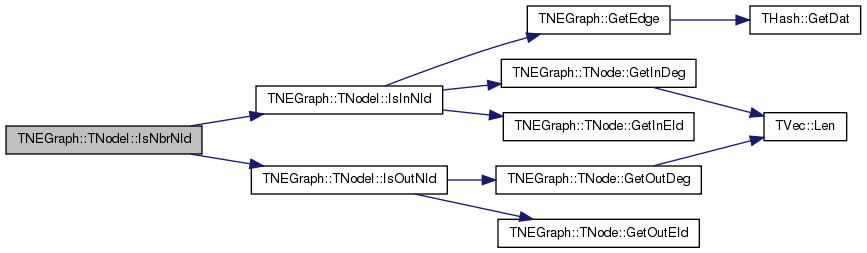bool TNEGraph::TNodeI::IsOutEId ( const int & EId ) const
inline

Tests whether the edge with ID EId is an out-edge of current node.

Definition at line 760 of file graph.h.

Referenced by IsNbrEId().

760 { return NodeHI.GetDat().IsOutEId(EId); }
THashIter NodeHI
Definition: graph.h:709

Here is the caller graph for this function: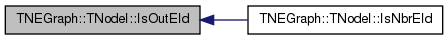bool TNEGraph::TNodeI::IsOutNId ( const int & NId ) const

Tests whether the current node points to node with ID NId.

Definition at line 477 of file graph.cpp.

References edge, TNEGraph::TNode::GetOutDeg(), and TNEGraph::TNode::GetOutEId().

Referenced by IsNbrNId().

477  {
478  const TNode& Node = NodeHI.GetDat();
479  for (int edge = 0; edge < Node.GetOutDeg(); edge++) {
480  if (NId == Graph->GetEdge(Node.GetOutEId(edge)).GetDstNId())
481  return true;
482  }
483  return false;
484 }
THashIter NodeHI
Definition: graph.h:709
TEdge & GetEdge(const int &EId)
Definition: graph.h:792
const TNEGraph * Graph
Definition: graph.h:710

Here is the call graph for this function: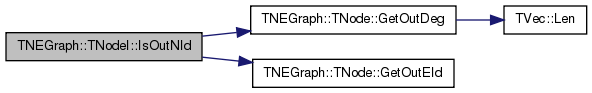Here is the caller graph for this function: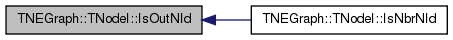TNodeI& TNEGraph::TNodeI::operator++ ( int )
inline

Increment iterator.

Definition at line 717 of file graph.h.

717 { NodeHI++; return *this; }
THashIter NodeHI
Definition: graph.h:709
 TNodeI& TNEGraph::TNodeI::operator-- ( int )
inline

Decrement iterator.

Definition at line 719 of file graph.h.

719 { NodeHI--; return *this; }
THashIter NodeHI
Definition: graph.h:709
 bool TNEGraph::TNodeI::operator< ( const TNodeI & NodeI ) const
inline

Definition at line 721 of file graph.h.

References NodeHI.

721 { return NodeHI < NodeI.NodeHI; }
THashIter NodeHI
Definition: graph.h:709
 TNodeI& TNEGraph::TNodeI::operator= ( const TNodeI & NodeI )
inline

Definition at line 715 of file graph.h.

References Graph, and NodeHI.

715 { NodeHI = NodeI.NodeHI; Graph=NodeI.Graph; return *this; }
THashIter NodeHI
Definition: graph.h:709
const TNEGraph * Graph
Definition: graph.h:710
 bool TNEGraph::TNodeI::operator== ( const TNodeI & NodeI ) const
inline

Definition at line 722 of file graph.h.

References NodeHI.

722 { return NodeHI == NodeI.NodeHI; }
THashIter NodeHI
Definition: graph.h:709

## Friends And Related Function Documentation

 friend class TNEGraph
friend

Definition at line 763 of file graph.h.

## Member Data Documentation

 const TNEGraph* TNEGraph::TNodeI::Graph
private

Definition at line 710 of file graph.h.

Referenced by IsInNId(), and operator=().

 THashIter TNEGraph::TNodeI::NodeHI
private

Definition at line 709 of file graph.h.

Referenced by IsInNId(), operator<(), operator=(), and operator==().

The documentation for this class was generated from the following files: